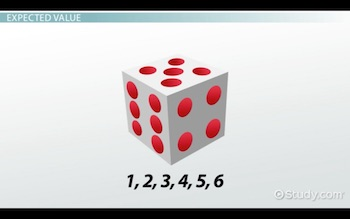# Finding & Interpreting the Expected Value of a Discrete Random Variable

Lesson Transcript
Instructor: Cathryn Jackson

Cat has taught a variety of subjects, including communications, mathematics, and technology. Cat has a master's degree in education and is currently working on her Ph.D.

Discrete random variables appear in your life a lot more than you think. You can use expected values to find the probability of a discrete random variable, as shown in this lesson. Updated: 10/31/2019

## Understanding Discrete Variables

Brady is playing a game with his friends. He needs to roll a 4 to avoid any bad things from happening to him in the game. He has two chances to roll a 4. Before he rolls the die, his friends bet if he is going to make it.

''Never gonna happen,'' says one.
''He's got a 50% chance!'' says another.
''You're wrong!'' says a third friend.

So what are Brady's chances of rolling a 4? Are any of his friends right?

In this lesson, you'll figure out Brady's chances of rolling a 4 by understanding discrete variables and learning how to use a formula to find the expected value of a discrete variable. First, let's define discrete random variables.

A discrete variable is an outcome of discrete data, which is data that cannot be divided; it is distinct and can only occur in certain values. In other words, a discrete variable is an experimental result that cannot be divided. For example, if you were to count the number of people in a classroom you would have a discrete variable because you can only have a whole person, not a half or a quarter of a person.

Brady's variable is discrete because he can only roll whole numbers with the die. We can also say that Brady's variable is random because he has no control over how the die will land. Experiments such as drawing a card from a deck, flipping a coin, or rolling a die are all examples of discrete random variables.

Now, let's talk about how you can predict the probability of a discrete random variable using a concept called expected value.

An error occurred trying to load this video.

Try refreshing the page, or contact customer support.

Coming up next: Developing Discrete Probability Distributions Theoretically & Finding Expected Values

### You're on a roll. Keep up the good work!

Replay
Your next lesson will play in 10 seconds
• 0:50 Understanding Discrete…
• 2:00 Definition of Expected Value
• 4:26 Lesson Summary
Save Save

Want to watch this again later?

Log in or sign up to add this lesson to a Custom Course.

Timeline
Autoplay
Autoplay
Speed Speed

## Expected Value

This is a 6-sided die:Each number (1, 2, 3, 4, 5, and 6) is represented on one side of the die. There is only one number on each side. These are important facts to keep in mind when finding the expected value of a discrete random variable. An expected value is simply the number of successful outcomes expected in an experiment. In Brady's case, the expected value is the probability that he will roll a 4 in two tries. Note that this is how you calculate probability or what you expect to happen, not necessarily the actual result of the experiment.

The formula for expected value of a discrete random variable is n * P. This is also considered the mean or average probability. The n represents the number of trials, and the P represents the probability of success on an individual trial. Before we solve Brady's problem, let's look at a different example to help you understand expected values.

To unlock this lesson you must be a Study.com Member.
Create your account

### Register to view this lesson

Are you a student or a teacher?

### Unlock Your Education

#### See for yourself why 30 million people use Study.com

##### Become a Study.com member and start learning now.
Back
What teachers are saying about Study.com
Create an account to start this course today
Used by over 30 million students worldwide